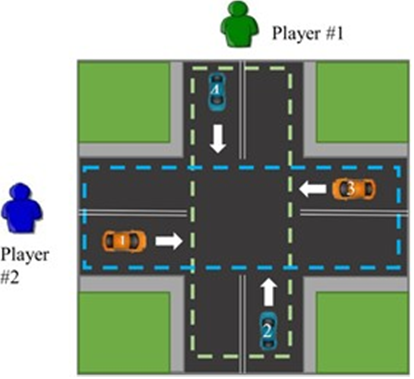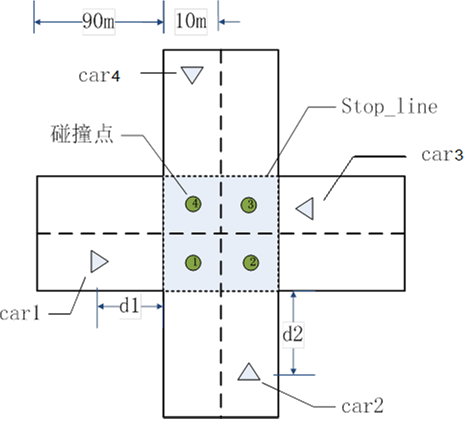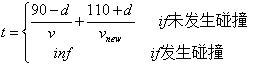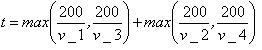www.b2bchain.cn

# 博弈论（二）： 车联网环境下一个基于 博弈论的十字路口智能 交通控制算法的讲解

• 问题描述

1、胆小鬼博弈{ (1,1), (1,0) , (0,1) , (1, −1) , (−1,1), (0,0) , (0, −1) , (−1,0) , (−1, −1) } ，例如

#1的动作为(1,0)代表他命令 2 号车加速，3 号车保持当前速度行驶。

1. 在给定车辆数据与玩家策略后可以运行驾驶模拟3.1数据：

3.2根据车辆动作速度更新：

3.3碰撞判定：

3.4博弈损失（通行时间）4.1计算博弈损失矩阵

 (1, 1) (1, 0) (1, -1) (0, 1) (0, 0) (0, -1) (-1, 1) (-1, 0) (-1, -1) (1, 1) (1, 0) (1, -1) (0, 1) (0, 0) (0, -1) (-1, 1) (-1, 0) (-1, -1)

Player #1 采取的策略 ：

Player #2 采取的策略 ：

4.2与传统方法对比• 实验结果

 (1, 1) (1, 0) (1, -1) (0, 1) (0, 0) (0, -1) (-1, 1) (-1, 0) (-1, -1) (1, 1) inf inf inf inf inf inf inf inf inf (1, 0) inf inf inf inf inf inf inf inf inf (1, -1) inf inf 7.3 inf inf 7.7 inf inf inf (0, 1) inf inf inf inf inf inf inf inf inf (0, 0) inf inf inf inf inf inf inf inf inf (0, -1) inf inf 7.5 inf inf 7.9 inf inf inf (-1, 1) inf inf inf inf inf inf inf inf inf (-1, 0) inf inf inf inf inf inf inf inf inf (-1, -1) inf inf inf inf inf inf inf inf inf

Player #1 采取的策略 ：[1, -1]

Player #2 采取的策略 ：[1, -1]

 最短通行时间 传统时间 1 [[-1, 1], [1, 1]] 8.04 18.85 2 [[1, 1], [0, 0]] 6.34 16.53 3 [[0, -1], [1, -1]] 8.38 14.84 4 [[0, 0], [0, 1]] 6.56 15.90 5 [[-1, 0], [1, 1]] 6.23 13.52 6 [[1, 1], [0, -1]] 7.57 17.08 7 All accident 17.25 8 All accident 15.90 9 [[-1, 0], [1, 1]] 6.20 14.16 10 All accident 11.78
• 代码展示
` `import random # 初始化一辆车 def init():     v = random.uniform(20, 40)     d = random.uniform(0, 90)     return [d, v, 0]  def cal_time(car):     return (90-car)/car + (110+car)/car  def tradition(car):     car1, car2, car3, car4 = car     return max(200/car1, 200/car3) + max(200/car2, 200/car4)  def game_run(player1, player2, car):     # 初始化车辆     car1, car2, car3, car4 = car     # 速度更新     car1 = car1 * (1 + player2 * 0.4)     car2 = car2 * (1 + player1 * 0.4)     car3 = car3 * (1 + player2 * 0.4)     car4 = car4 * (1 + player1 * 0.4)     # 碰撞分析     accident = any([abs((car4 + 15) / car4 - (car1 + 5) / car1) < 1,                   abs((car1 + 15) / car1 - (car2 + 5) / car2) < 1,             			  abs((car2 + 15) / car2 - (car3 + 5) / car3) < 1,                   abs((car3 + 15) / car3 - (car4 + 5) / car4) < 1])        return (cal_time(car1) + cal_time(car2) + cal_time(car3) + cal_time(car4)) / 4 + accident * 9999999  def main():     # 设置玩家行动     # player1 = [1, 1]      # player2 = [1, 1]      action = [[1, 1], [1, 0], [1, -1], [0, 1], [0, 0], [0, -1], [-1, 1], [-1, 0], [-1, 1]]     car = [init(), init(), init(), init()]     print("init car:", car)     min_t = 999     min_t_action = None     for i in range(9):         for j in range(9):             t = game_run(action[i], action[j], car)  # run(player1, player2)             if t < 9999999:                 print("%.1f" % t, end=" ")                 if t < min_t:                     min_t = t                     min_t_action = [action[i], action[j]]             else:                 print("inf", end=" ")         print()     if min_t_action:         print("min of game: ", min_t_action, "%.1f" % min_t)     else:         print("min of game: accident")     print("tradition time: ", "%.1f" % tradition(car))   if __name__ == "__main__":     main()``

• 微信咨询
• 回顶